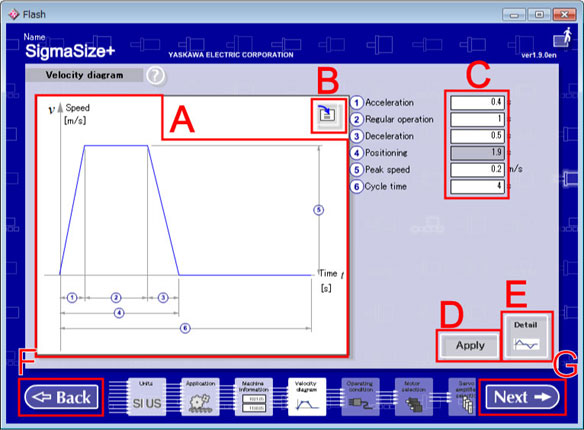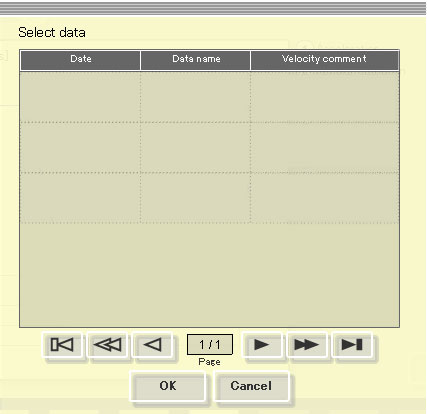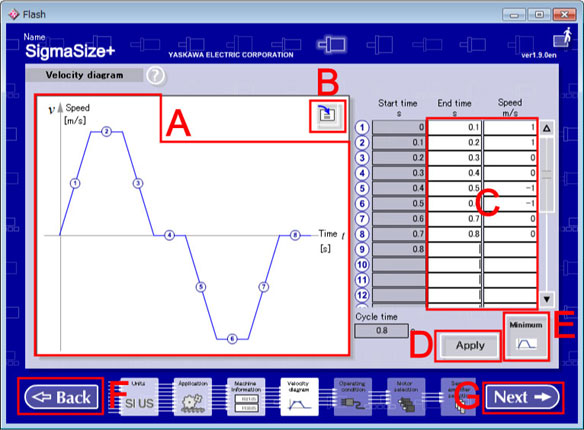Wizard sizing / Velocity diagram

Velocity diagram

Input a reference speed. There are two input methods. One is minimum input and the other is detailed input.
It is the minimum input in initial state, default setting value and its velocity diagram are displayed.
When data were load with Data load/ delete, or Velocity diagram was already set, the Velocity diagram that was already finished setting are displayed.

Details for the Minimum Input and Detailed Input are shown below.

[Operation Procedure]

1. Input data in the data input column.
2. Click "Apply" to redraw a velocity diagram.
3. Click "Next" to advance to the Operating condition.

*Relation between moving direction of load and sign of velocity
With Machine Information a right direction or a load's movement of a upper direction are defined as a plus.
In other word, if you input a plus value at a column of speed with "Velocity diagram", a load moves to right direction or upper direction.

[Screen Structure]Velocity diagram Screen (Minimum Input)

1. Velocity diagram Preview
Displays the set velocity diagram of a reference speed.
In an initial status, default values are displayed.
Units of each axis are displayed units were set with Unit.

Loads a reference speed value from saved data.
The loaded data is converted to the current setting unit.
The converted data may be affected according to the roundoff error in unit conversion.
The loaded data may differ because it rounds off by the 4th place of the decimal point of index expression when unit conversion are carried out.1. Click the Data Load button.
Data is displayed from the latest one at the date.
When the target data is not displayed, click a Page Move button.
2. Select a data for loading, and then, click a [OK] button.

3. Data Input Column
Set a speed reference value.
Input items are different by selected application.
In addition, units of each item in the columns are displayed units were set with Unit.

In an initial status, default values are displayed.

When you selected anything other than vertical application with Application or loaded the data that is selected anything other than vertical application with "Velocity diagram".
 Item Explanation Range which can be inputted Acceleration Display/Input acceleration time. Larger than 0 and less than 1.0e+025 Regular operation Display/Input regular operating time. 0 or more and less than 1.0e+025 Deceleration Display/Input deceleration time. Larger than 0 and less than 1.0e+025 Positioning Calc and Display positioning time. Automatically calculated in re-drawing. Peak speed Display/Input peak time. Larger than 0 and less than 1.0e+025 Cycle time Display/Input cycle time. Larger than 0 and less than 1.0e+025

When a vertical mechanism is selected on the machine information screen or
load a data for vertical mechanism on the velocity diagram.
 Item Explanation Range which can be inputted Normal acceleration Display/Input Normal acceleration time. Larger than 0 and less than 1.0e+025 Regular operation Display/Input regular operation time. 0 or more and less than 1.0e+025 Normal deceleration Display/Input Normal deceleration time. Larger than 0 and less than 1.0e+025 Normal positioning Calc and Display Normal positioning time. Automatically calculated in re-drawing. Normal peak speed Display/Input Normal positioning time. Larger than 0 and less than 1.0e+025 Normal cycle time Display/Input Normal cycle time. Larger than 0 and less than 1.0e+025 Reverse acceleration Display/Input Reverse acceleration time. Larger than 0 and less than 1.0e+025 Reverse regular operation Display/Input Reverse regular operation time. 0 or more and less than 1.0e+025 Reverse deceleration Display/Input Reverse deceleration time. Larger than 0 and less than 1.0e+025 Reverse positioning Calc and Display Reverse positioning time. Automatically calculated in re-drawing. Reverse peak speed Display/Input Reverse peak speed. Larger than -1.0e+025 and smaller than 0 Reverse cycle time Display/Input Reverse cycle time. Larger than 0 and less than 1.0e+025

The number of maximum input beams of each input column is 12 figures(include such as a mark and a decimal point).
Example
Possible:   :1.0,-1.0,1.2345678912,-1.234567891,1.0e+100   etc
Impossible:1.00000000000,-1000000000,1.23456789123,1.000000e+001   etc

Limit which can be inputted
ItemLimit
Regular operation time
Positive regular operation time
negative regular operation time
0 or more and less than 1.0e+025
OthersLarger than 0 and less than 1.0e+025

The number of maximum input beams of each input column is 12 figures(include such as a mark and a decimal point).
Example
Possible  :1.0,-1.0,1.2345678912,-1.234567891,1.0e+100  etc.
Impossible;:1.00000000000,-1000000000,1.23456789123,1.000000e+001  etc.

4. Apply button
Verifies input value and redraws a velocity diagram.

5. Detailed Input button
Switches input method to the "Detailed Input".

6. Back button
Returns to the Machine information.

7. Next button
Checks input data, and then advances to the Operating condition.

[Operation Procedure]

1. Enter an end time and attainment speed, and then click "Apply".
Cannot input a blank or the numerical value out of range.
2. Goes to the Minimum input by click the Minimum button.
3. Advance to the Operating condition by clicking "Next".

[Screen Structure]Velocity diagram Screen (Detailed Input)

1. Velocity Diagram Preview
Displays a set velocity diagram of a reference speed.
In an initial status, default values are displayed.
Units of each axis are displayed units were set with Unit.

Refer to Minimum Input

3. Data Input Column
Enter an end time and attainment speed.
The start time and cycle time is automatically calculated.
Input up to 40 digits is allowed.

When data is read or speed instructions are already set up, the value at that time is displayed.
Decide inputted values and advances to the Operation condition to click [Next].

* When input velocity from positive to negative (negative to positive), input 0 between positive and negative (negative and positive).

4. Apply button
Verifies the input value and redraws a velocity diagram.

5. Minimum Input button
Switches input method to the Minimum Input.

6. Back button
Returns to the Machine information.

7. Next button
Checks input data, and then advances to the Operating condition.